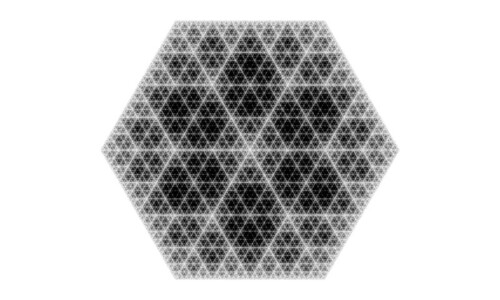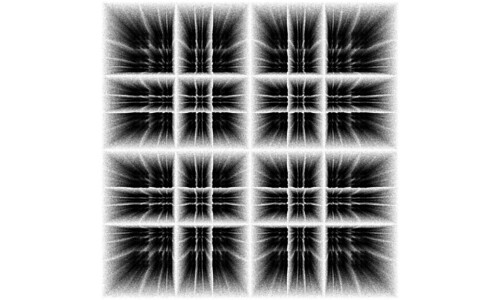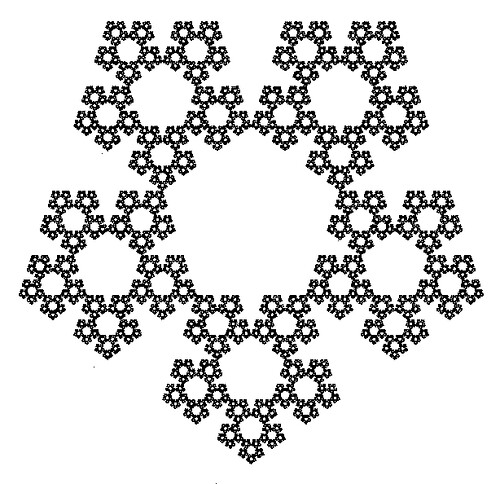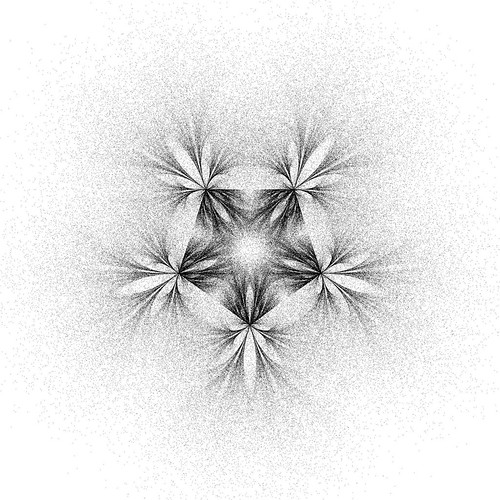## Chaos Game and the Hexagon

For a hexagon the chaos game forms the same pattern at 1.5 as it does 0.5.Posted in Uncategorized | 1 Comment

## The Chaos Game and PHI

The outer chaos game shows some interesting patterns with phi as a magnitude.

## 3D Shapes Painted by Sections of the Outer Chaos Game

Square with magnitude range 1.4-1.5:Pentagon with magnitude range 1.3-1.4:## Personal Website

I have setup a personal website koozdra.ca.

There is a preliminary demo of the Chaos Game Path Plotter.

http://koozdra.ca/chaosgame/main.html

## More Interesting Patterns Hidden in the Chaos Game

UPDATE: Interactive version Chaos Game

I was curious to see what would happen If generated chaos games in ascending magnitudes and drew them together. Typically a pattern emerges from 0.5 to 1.5 magnitudes depending on the number of attractors. I split up the spectrum into two parts 0.5 to 1 and 1 to 1.5. This is done because values above 1 draw into the middle of the attractors. This interferes with the internal pattern.

## 175

Posted in Uncategorized | 7 Comments

## 1.5 Magnitude Pentagon Chaos Game

If at each iteration in the chaos game the current point is moved 1.5 times the distance to a randomly selected pentagonal vertex attractor, this image is created.This image seemed familiar to me. I had created a magnetic shape that looked very similar.## Adding a Little More Chaos to the Chaos Game

The Chaos Game defines a set of attractors and an iterative process that moves a point towards a random attractor by a specified magnitude.

This image is generated by having five attactors, the points of a pentagon. At each iteration the current point moves towards a point on the pentagon by PHI (0.618) times the distance to the attractor.I wanted to see what would happen if the magnitude of attraction was also chaotic. At each stage of the algorithm a random attraction magnitude is chosen between [0.5,1.5].More Resources:
Mathworld Chaos Game Page
Wikipedia Chaos Game Page

[Update] Processing code to generate the shapes above:
``` var attractors = []; var current = [random(800),random(800)]```

``` for(var i = 0; i < 6; i++){ attractors.push([cos(i * 2 * PI/5) * 300, sin(i * 2 * PI/5) * 300]); } var attraction = 0.5; void draw(){ translate(width/2, height/2); rotate(PI/2); stroke(0); for(var i = 0; i < attractors.length; i++){ rect(attractors, attractors, 5,5); } for(var i = 0; i < 100; i++){ point(current,current); var target = attractors[Math.floor(Math.random() * (attractors.length-1))] current = current - (current - target) * attraction; current = current - (current - target) * attraction; } attraction = Math.random()+.5; ```

```} ```

Posted in Uncategorized | Tagged , , | 7 Comments